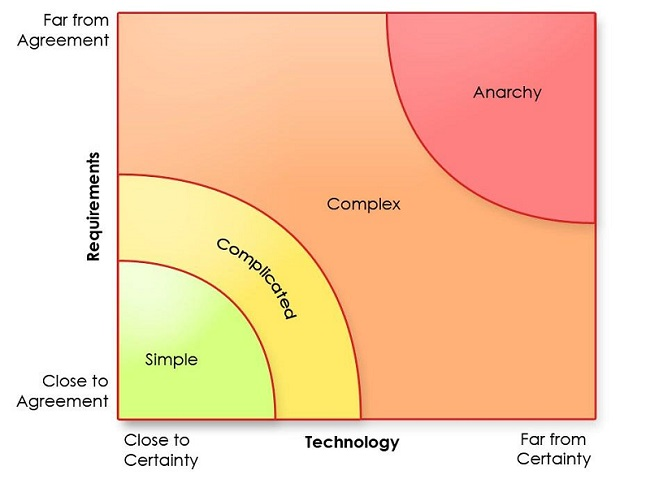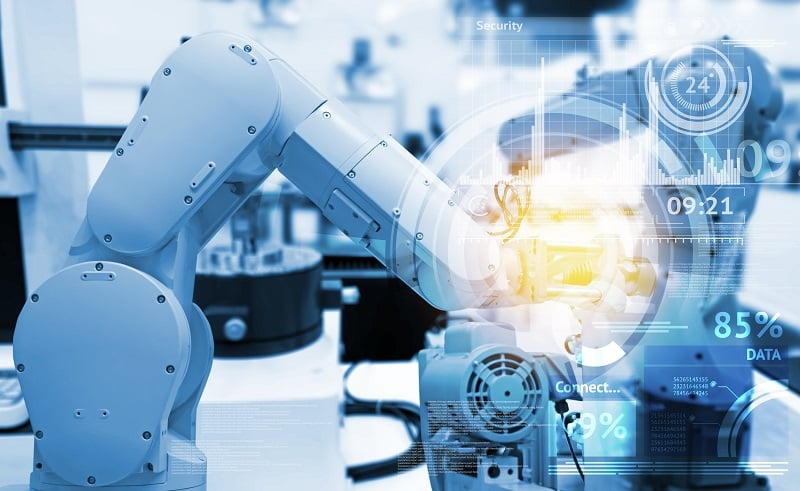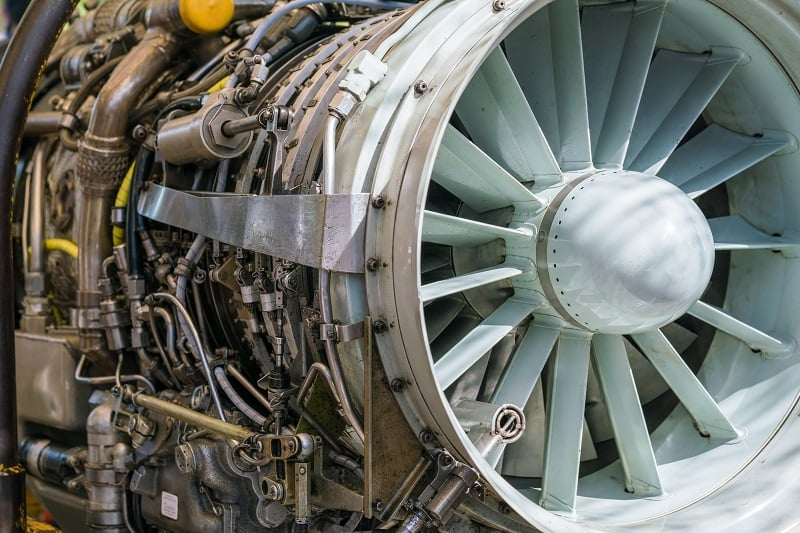Technical Article

# Introduction to System Dynamic Responses

May 24, 2023 by Antonio Armenta

## System dynamics is primarily concerned with understanding complex systems’ behaviors over time. Mathematical and computer models can help identify responses before turning to costly real-world experiments.

The word “system” is among the most utilized in engineering. But have you ever stopped to think about its meaning? What is a “system”?

In the most basic definition, systems are collections of elements that follow a set of rules, creating a unified whole. Endless examples of systems can be found around us, but they all have these things in common.

Systems in control engineering are no different. In this article, we will walk through the basics of elements and rules from the point of view of control engineering. This study of these elements and rules is also known as system dynamics.### System Dynamics: Studying Complex Systems

System dynamics is a field of engineering that focuses on studying complex systems. Complexity, like system, is another popular word whose meaning can be deduced. But, in control theory, complexity does, in fact, have a concise definition.

There are four types of systems under the lens of control theory: simple, complicated, complex, and chaotic. A simple system has a comparatively small number of elements and/or subsystems. The relationships or rules that exist between its elements are understood and, thus, produce a predictable result. There is only one path to arrive at the solution.

Complicated systems are the next level. The rules of these systems offer multiple paths to arrive at the solution. It may take more work (or computer processing), but a complicated system's elements and rules can still be understood and predicted.

A complex system, like a complicated one, also has many elements and rules. The key difference between them is a degree of unpredictability. Complex systems consist of unknowns that can only be evaluated once something actually happens. That is, when there is an input to the system, we see the resulting output. We can formulate a hypothesis about the output, but a complex system can produce multiple solutions.##### Figure 2. The Stacey complexity model. Image used courtesy of scrum-tips

Although the Stacey complexity model above was created to evaluate the complexity of tasks and processes, it can help us understand systems when we view technology as the level of certainty and requirements as the rules of the system.

At the top right corner of the graph is anarchy, which best describes chaotic systems. These systems are highly unpredictable, and studying them necessitated the creation of a specialized field called chaos theory.

### Electromechanical Complex Systems

Let us take a few steps back and concentrate on complex systems—particularly electrical and mechanical complex systems–which will bring us closer to control automation. Purely electrical, purely mechanical, and electromechanical systems comprise some of the most common elements we are familiar with. The concepts of mass, displacement, velocity, acceleration, damping, and spring coefficient are crucial for modeling and understanding these systems.##### Figure 3. Robots are complex systems with a relatively low degree of uncertainty. Image used courtesy of Adobe Stock

System dynamics is primarily concerned with understanding complex systems’ behaviors over time. As previously discussed, these systems have a considerable degree of uncertainty. Computer models are essential to evaluating complex systems because the unknowns need to be tested to prove a hypothesis. However, evaluating theories with real-life systems can be highly impractical and costly.

Take, for example, a complex hydraulic system at risk of being exposed to harsh environmental conditions. Hundreds or even thousands of elements, including actuators, selector valves, check valves, accumulators, and filters interconnected by an intricate network of pipes of different gauges. Pressure and fluid velocity are crucial variables to control. Now, situate this already intrinsically complex system and subject it to changing external environmental conditions. We are actually talking about most aircraft systems.##### Figure 4. Aircraft hydraulic systems can be highly complex. Image used courtesy of Adobe Stock

Computer models and simulations of aircraft hydraulic systems have been vital in identifying design issues and making system improvements before mass production. In a computer simulation like this, one of the main goals is to analyze the system’s outputs upon different inputs. For example, think of wind, friction, and outside temperature as possible inputs to the simulation of this complex system.

### System Dynamics Response

Once we have built a complex system and its rules, we want to understand how it can change over time in response to different inputs. Therefore, the mathematical models that describe the system must include the relationships between inputs and outputs and the internal relationships of variables.

The fundamentals behind the mathematical models employed rely on the complex systems’ subtypes. For example, the standard equation of motion, or Newton’s Law, is the basis for most mechanical systems, while Ohm’s Law and Kirchhoff’s Law are essential in electrical systems.

The steady-state analysis is performed to introduce the time dimension in studying a complex dynamic system. Such an analysis involves identifying the smallest set of system variables (or elements) needed to understand the system's behavior upon a certain input. That set of variables is called state variables.